Math Calculators, Lessons and Formulas

It is time to solve your math problem

mathportal.org
• Geometry
• Circles
• Circle formulas

# Circle formulas

ans:
syntax error
C
DEL
ANS
±
(
)
÷
×
7
8
9
4
5
6
+
1
2
3
=
0
.
auto next question
calculator
•  Question 1: 1 pts Which expression can be used to find the area of a circle shown on the picture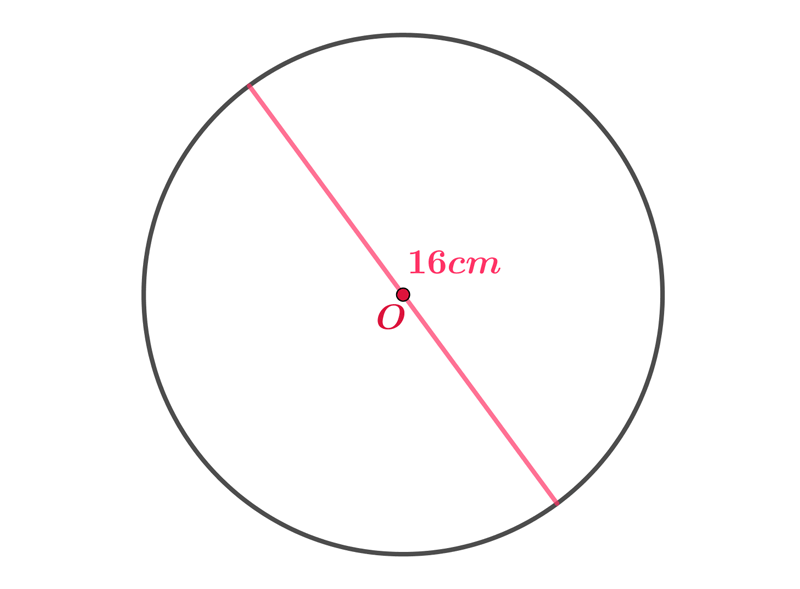$A=16\pi cm^{2}$ $A=2\cdot8\pi cm^{2}$ $A=2\cdot 8^{2}\pi cm^{2}$ $A=8^{2}\pi cm^{2}$
•  Question 2: 1 pts Which expression can be used to find the perimeter of a circle shown on the picture.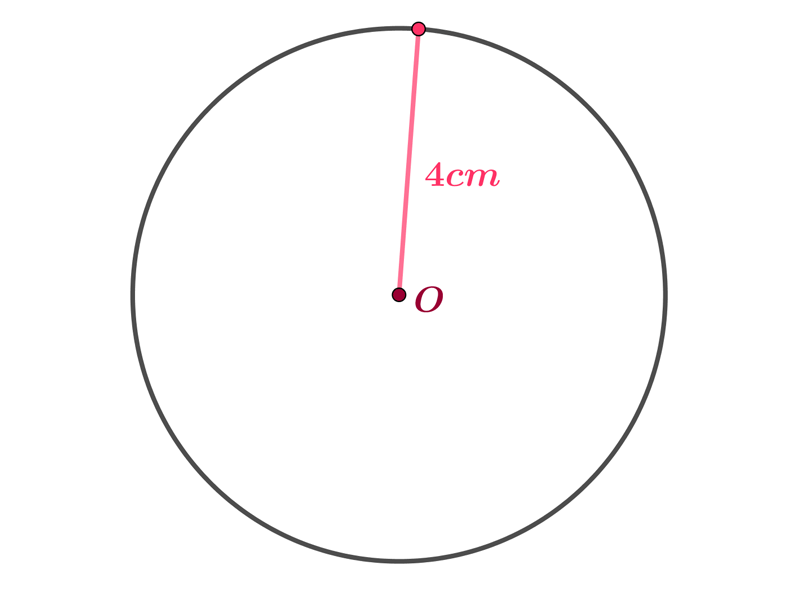$P=4\pi cm$ $P=2\cdot4\pi cm$ $P=2\cdot 4 cm$ $P=4^{2}\pi cm$
•  Question 3: 1 pts Find the arc length of an arc formed by $36^{\circ}$ of a circle with a radius of $5$ inches.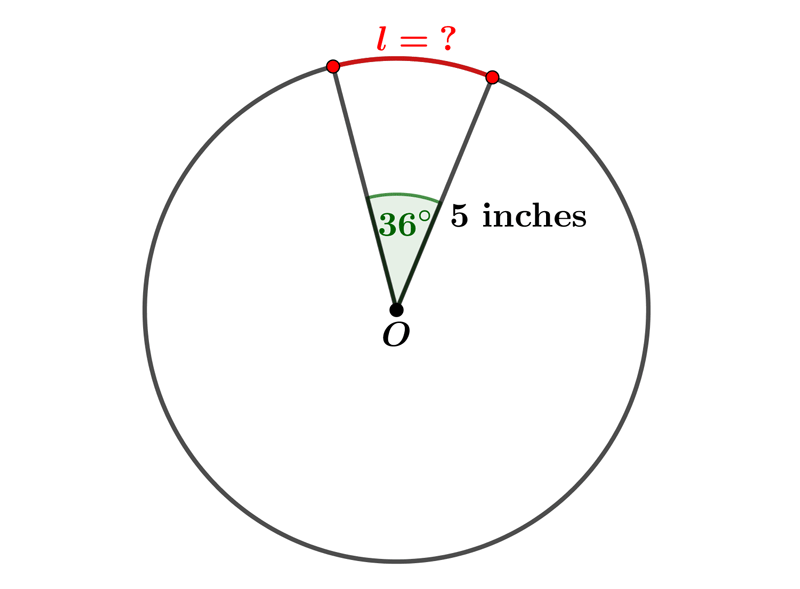$l=\dfrac{5^{2}\pi 36^{\circ}}{360^{\circ}}$ $l=\dfrac{10\pi 36^{\circ}}{360^{\circ}}$ $l=\dfrac{5\pi 36^{\circ}}{360^{\circ}}$ $l=\dfrac{10\pi 360^{\circ}}{36^{\circ}}$
•  Question 4: 1 pts Which expression can be used to find the area of a sector,$A_{s}$, shown on the picture.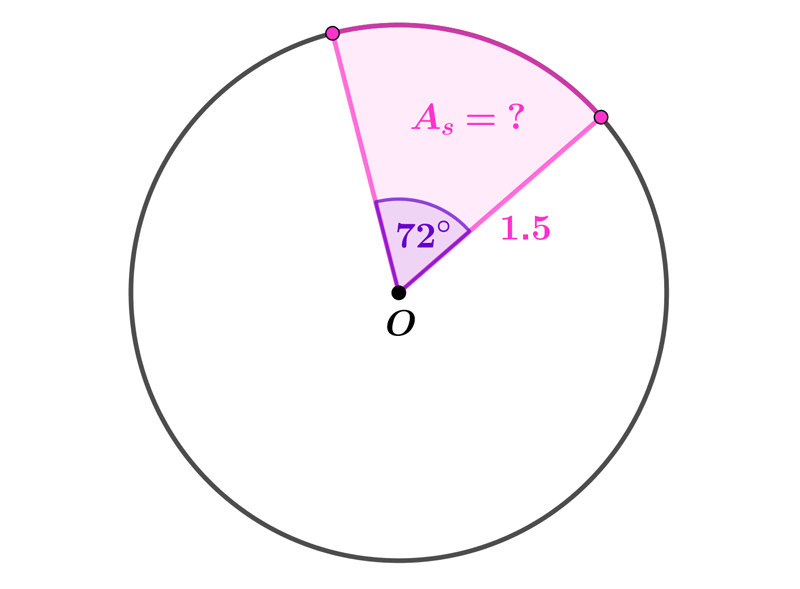$A_{s}=\dfrac{1.5\cdot \pi\cdot 72^{\circ}}{360^{\circ}}$ $A_{s}=\dfrac{(1.5)^{2}\cdot \pi\cdot 72^{\circ}}{180^{\circ}}$ $A_{s}=\dfrac{(1.5)^{2}\cdot \pi\cdot 72^{\circ}}{360^{\circ}}$ $A_{s}=\dfrac{1.5\cdot \pi\cdot 72^{\circ}}{180^{\circ}}$
•  Question 5: 2 pts The area of the sector is $18\pi cm^{2}$. Find the length of $x.$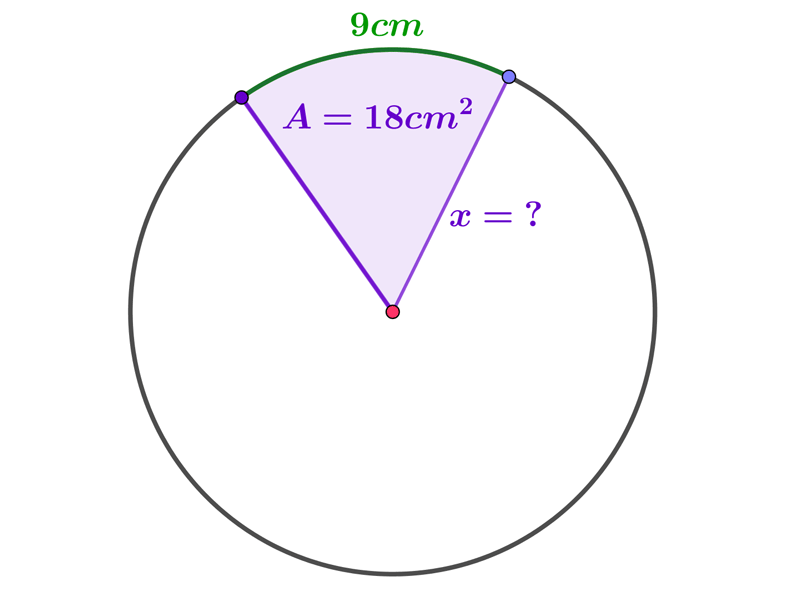$x=$
•  Question 6: 2 pts A path $8 m$ wide surrounds a circular lawn whose diameter is $110 m.$ Which expression can be used to find the area of the path.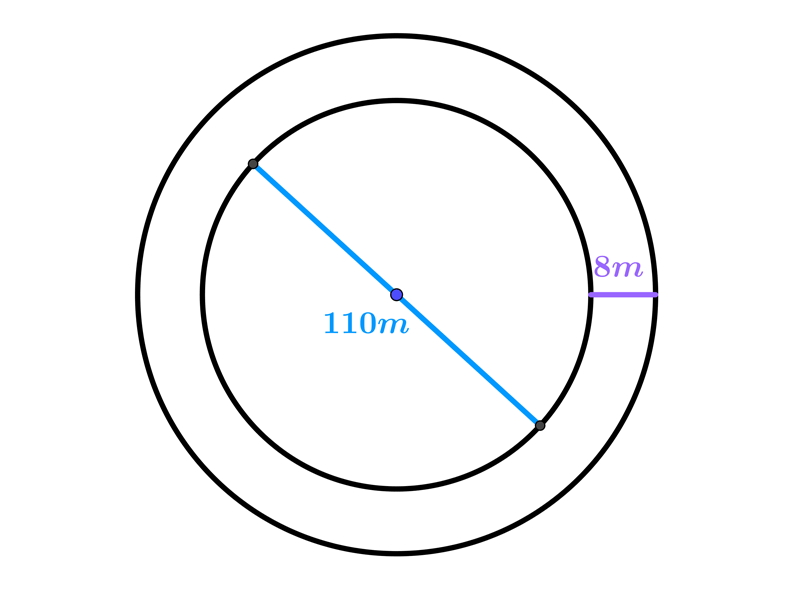$A=\pi \cdot\left(63^{2}-55^{2}\right)cm^{2}$ $A=\pi \cdot\left(55^{2}-8^{2}\right)cm^{2}$ $A=\pi \cdot\left(55^{2}-47^{2}\right)cm^{2}$ $A=\pi \cdot\left(60^{2}-55^{2}\right)cm^{2}$
•  Question 7: 2 pts Find the distance between the centres of the circles shown on the picture.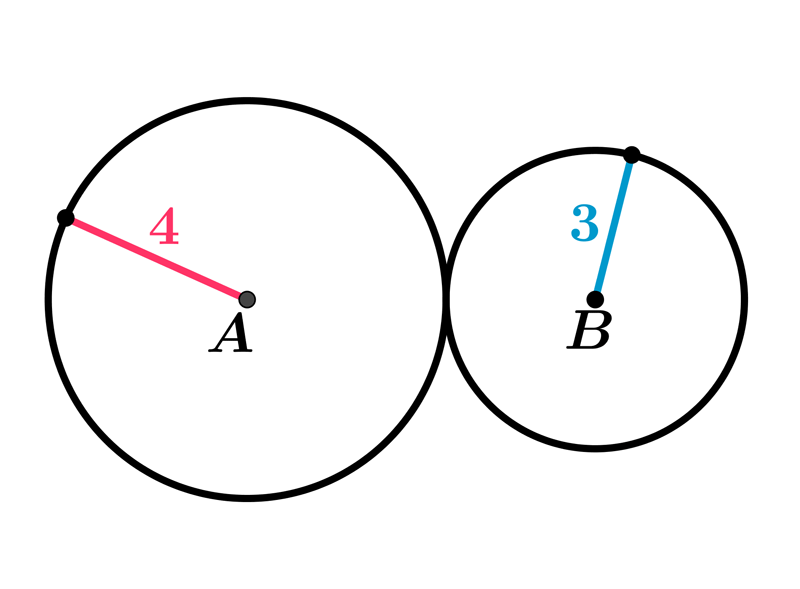•  Question 8: 2 pts Find the perimeter of the figure shown on the picture.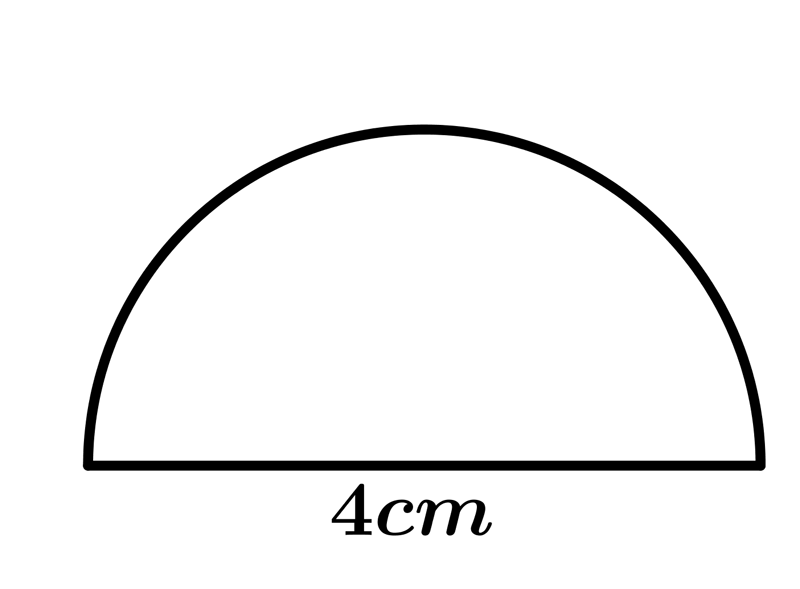$P=2\cdot(2+2\pi)cm$ $P=\pi \cdot(2+\pi)cm$ $P=2\cdot(1+\pi)cm$ $P=2\cdot(2+\pi)cm$
•  Question 9: 3 pts Find the area of the shaded figure shown on the picture, if the area of shown rectangle is $32cm^{2}$.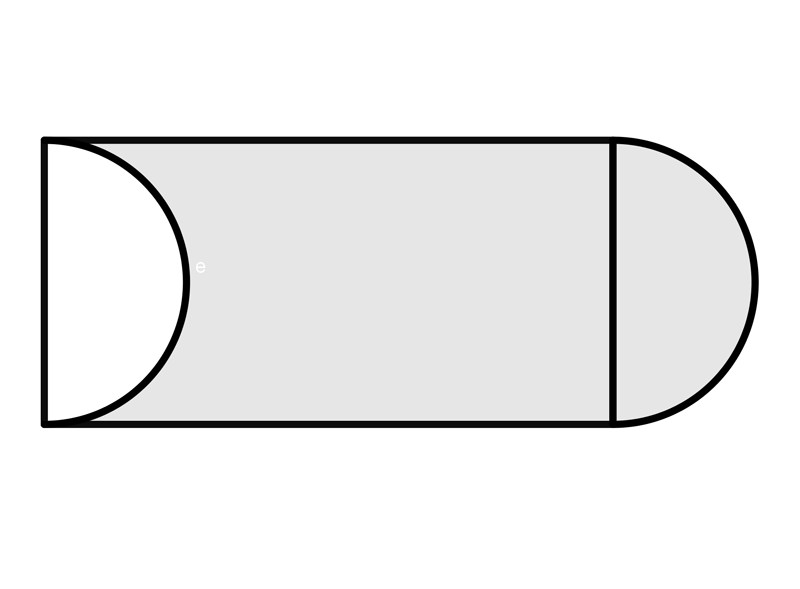$A=$
•  Question 10: 3 pts Find the perimeter of the shaded area shown on the picture.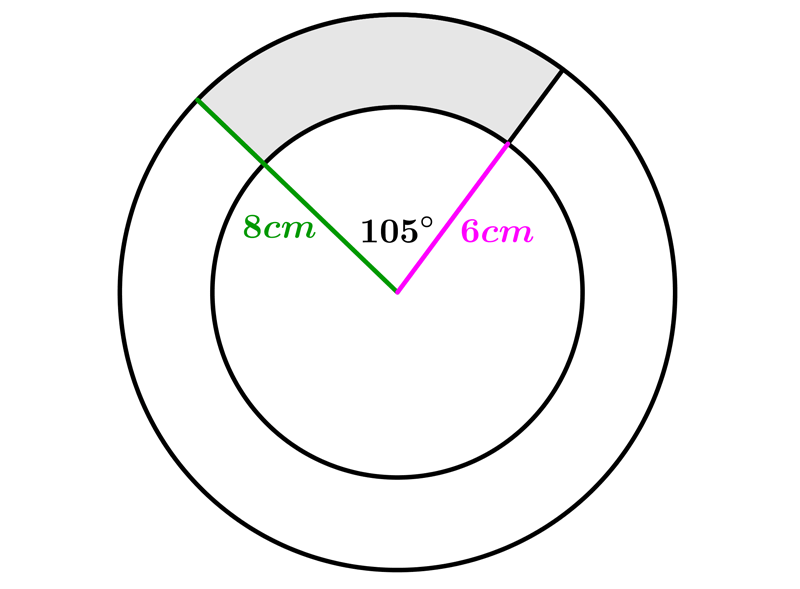$P=\dfrac{24+49\pi}{6}cm$ $P=\dfrac{25\pi}{6}cm$ $P=\dfrac{25+48\pi}{6}cm$ $P=\dfrac{49\pi}{6}cm$
•  Question 11: 3 pts Find the perimeter of the shaded area shown on the picture, if the radius of the biggest circle is $4cm.$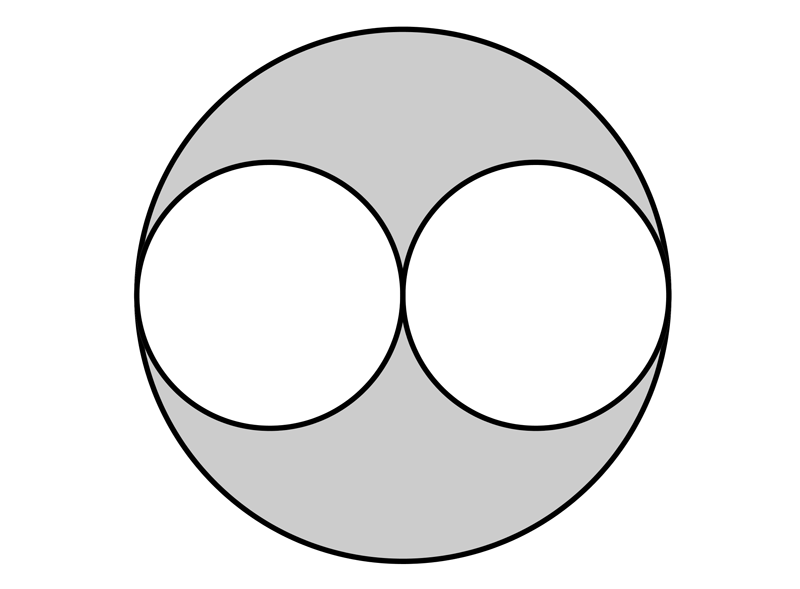$P=$
•  Question 12: 3 pts Find the perimeter of the shaded area shown on the picture.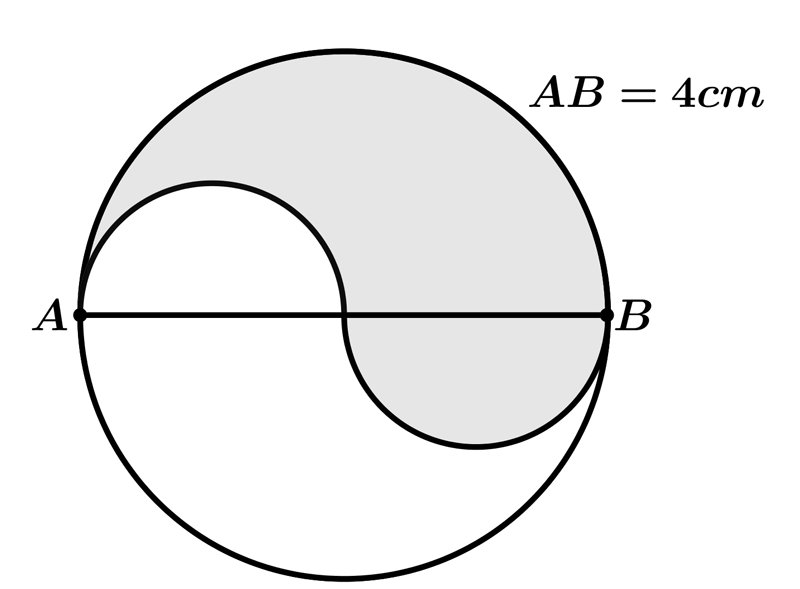$P=$